# Review n n n Unit contribution margin and

• Slides: 37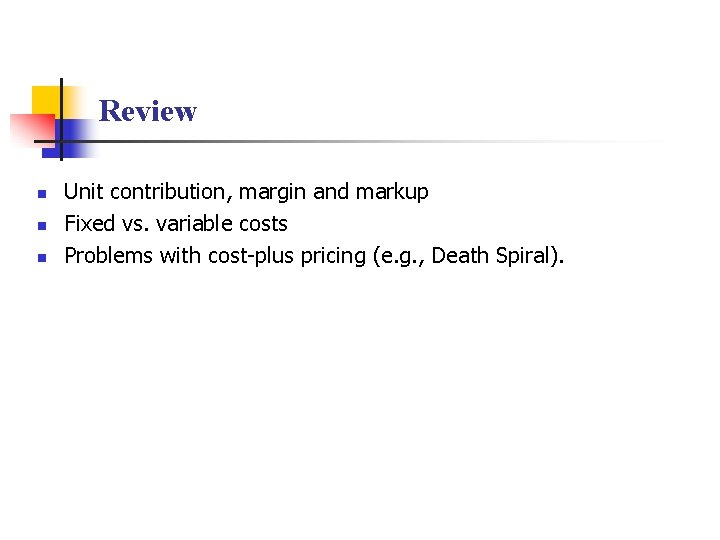Review n n n Unit contribution, margin and markup Fixed vs. variable costs Problems with cost-plus pricing (e. g. , Death Spiral).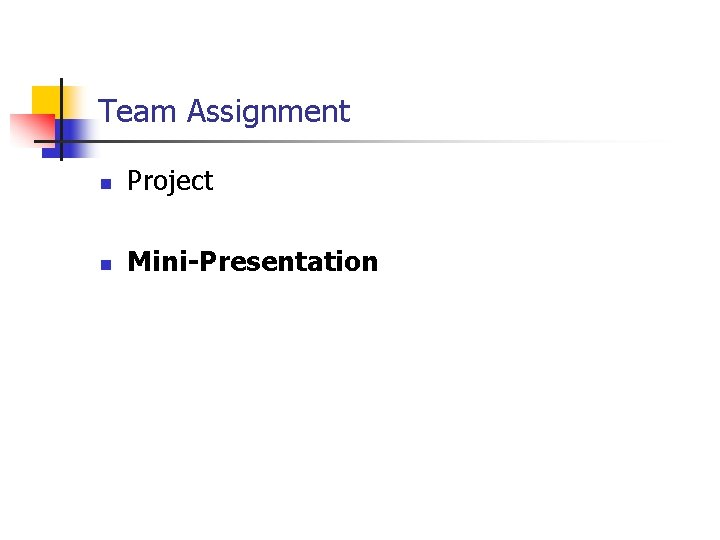Team Assignment n Project n Mini-PresentationProblems with Cost-plus Pricing n n Cost-plus pricing will lead to over-pricing in a weak market. Cost-plus pricing will lead to under-pricing in a strong market.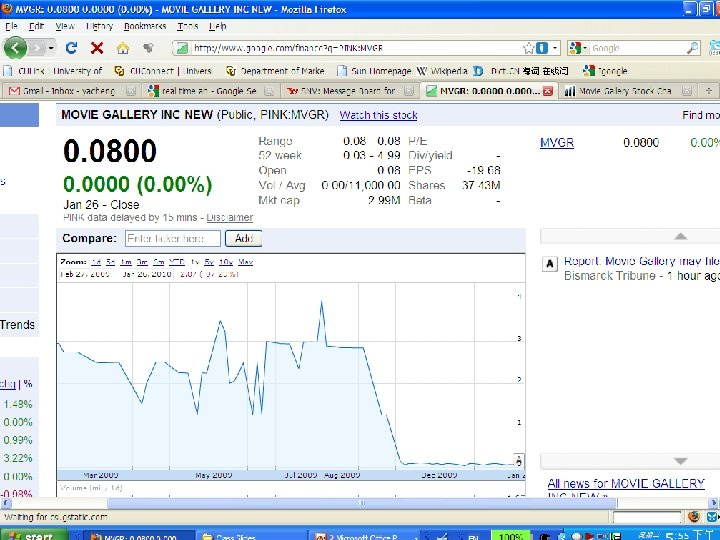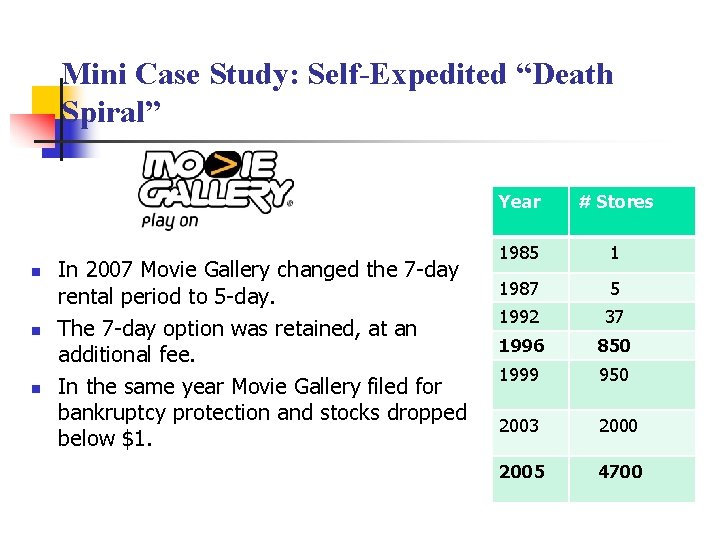Mini Case Study: Self-Expedited “Death Spiral” n n n In 2007 Movie Gallery changed the 7 -day rental period to 5 -day. The 7 -day option was retained, at an additional fee. In the same year Movie Gallery filed for bankruptcy protection and stocks dropped below \$1. Year # Stores 1985 1 1987 5 1992 37 1996 850 1999 950 2003 2000 2005 4700What Could Have MG Done? Channel strategy n n Pricing strategyPricing Based on Markup / Margin A retailer buys a sofa for \$1000. What will be the retailer’s selling price if it decides to go with (a) 30% markup and (b) 30% margin? n Markup n n n The \$ markup is \$1000*30%=\$300 Selling price = \$1000 + \$300 = \$1300 Margin n Selling price = p, say. The unit contribution is p*30/100 p = 1000 + 0. 3*p, so p=\$1428 7Chapter 9 Financial AnalysisTypes of BEP Type I: BEP of a price change Type II: BEP of a fixed-cost investment BEP Analysis Type III: BEP of the change in variable cost Type IV: BEP of CannibalizationTypes of BEP Type I: BEP of a price change Type II: BEP of a fixed-cost investment BEP Analysis Type III: BEP of the change in variable cost Type IV: BEP of Cannibalization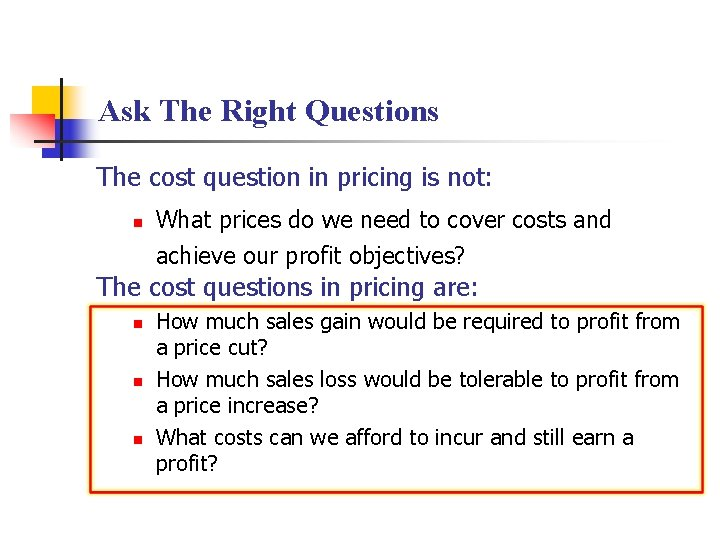Ask The Right Questions The cost question in pricing is not: n What prices do we need to cover costs and achieve our profit objectives? The cost questions in pricing are: n n n How much sales gain would be required to profit from a price cut? How much sales loss would be tolerable to profit from a price increase? What costs can we afford to incur and still earn a profit?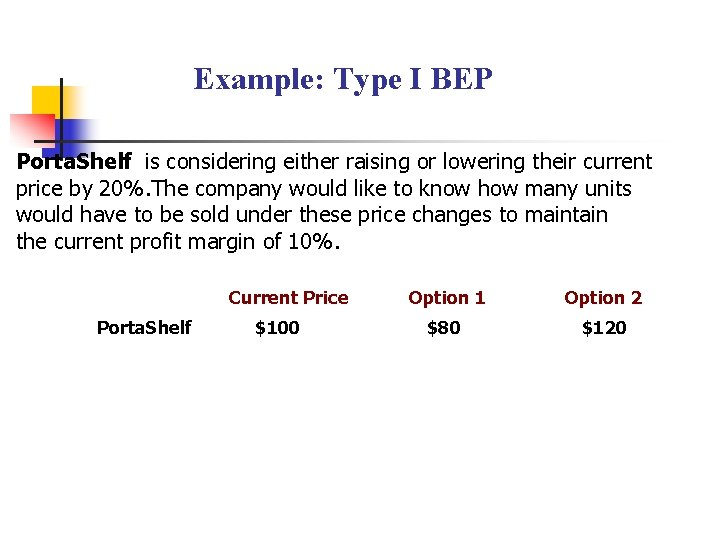Example: Type I BEP Porta. Shelf is considering either raising or lowering their current price by 20%. The company would like to know how many units would have to be sold under these price changes to maintain the current profit margin of 10%. Current Price Porta. Shelf \$100 Option 1 Option 2 \$80 \$120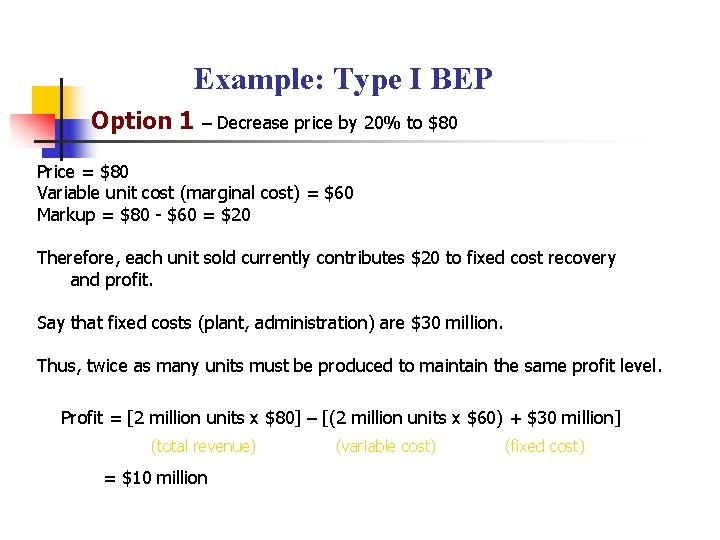Example: Type I BEP Option 1 – Decrease price by 20% to \$80 Price = \$80 Variable unit cost (marginal cost) = \$60 Markup = \$80 - \$60 = \$20 Therefore, each unit sold currently contributes \$20 to fixed cost recovery and profit. Say that fixed costs (plant, administration) are \$30 million. Thus, twice as many units must be produced to maintain the same profit level. Profit = [2 million units x \$80] – [(2 million units x \$60) + \$30 million] (total revenue) = \$10 million (variable cost) (fixed cost)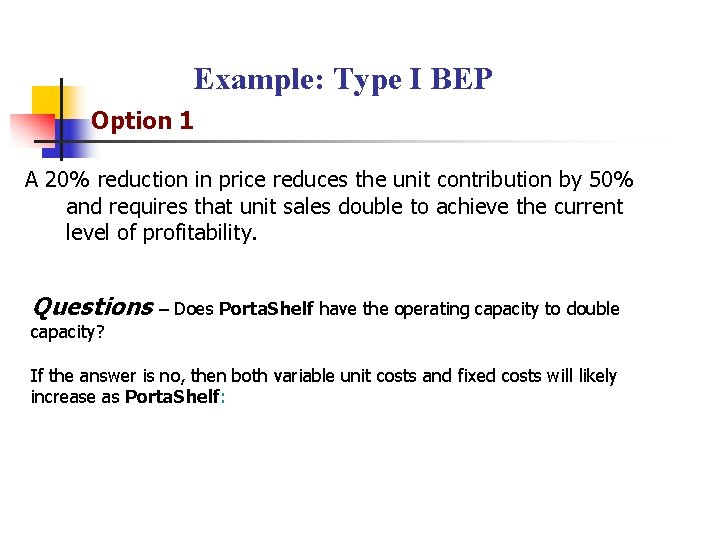Example: Type I BEP Option 1 A 20% reduction in price reduces the unit contribution by 50% and requires that unit sales double to achieve the current level of profitability. Questions – Does Porta. Shelf have the operating capacity to double capacity? If the answer is no, then both variable unit costs and fixed costs will likely increase as Porta. Shelf: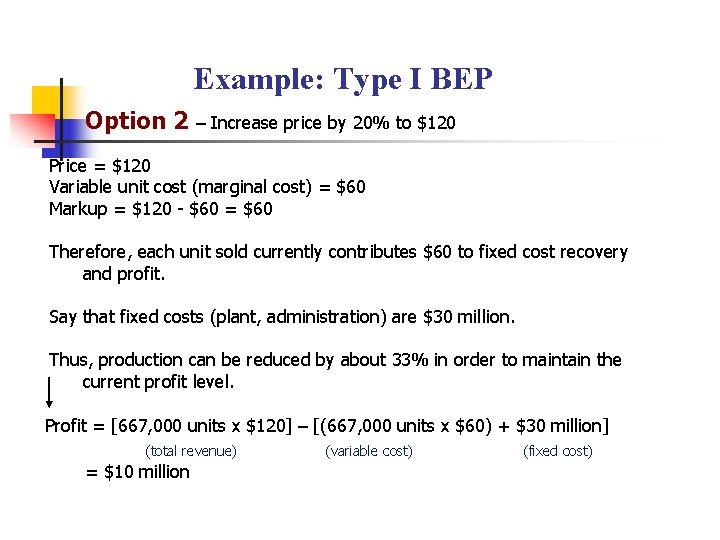Example: Type I BEP Option 2 – Increase price by 20% to \$120 Price = \$120 Variable unit cost (marginal cost) = \$60 Markup = \$120 - \$60 = \$60 Therefore, each unit sold currently contributes \$60 to fixed cost recovery and profit. Say that fixed costs (plant, administration) are \$30 million. Thus, production can be reduced by about 33% in order to maintain the current profit level. Profit = [667, 000 units x \$120] – [(667, 000 units x \$60) + \$30 million] (total revenue) = \$10 million (variable cost) (fixed cost)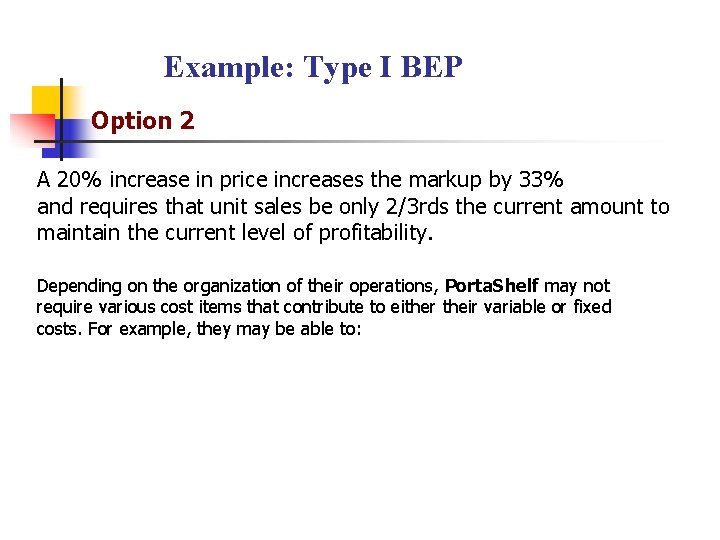Example: Type I BEP Option 2 A 20% increase in price increases the markup by 33% and requires that unit sales be only 2/3 rds the current amount to maintain the current level of profitability. Depending on the organization of their operations, Porta. Shelf may not require various cost items that contribute to either their variable or fixed costs. For example, they may be able to: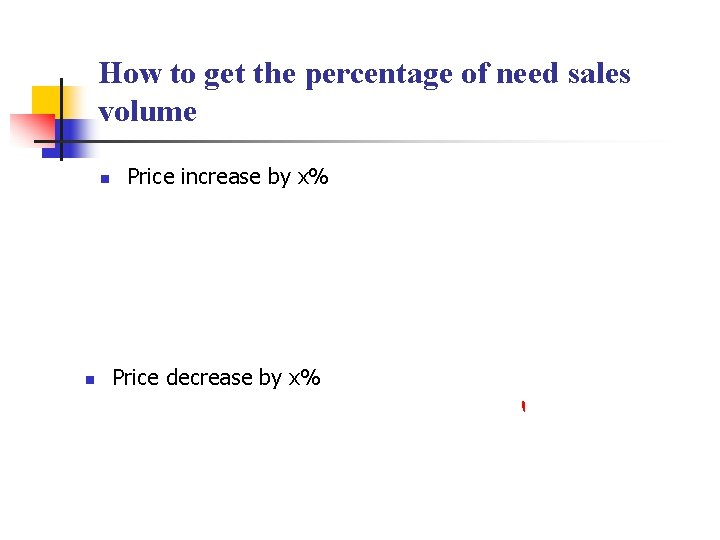How to get the percentage of need sales volume n n Price increase by x% Price decrease by x%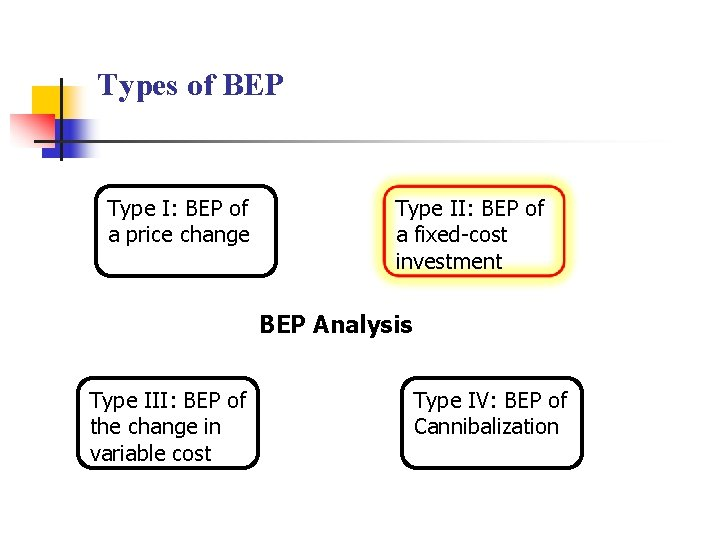Types of BEP Type I: BEP of a price change Type II: BEP of a fixed-cost investment BEP Analysis Type III: BEP of the change in variable cost Type IV: BEP of CannibalizationTotal, Variable, and Fixed Costs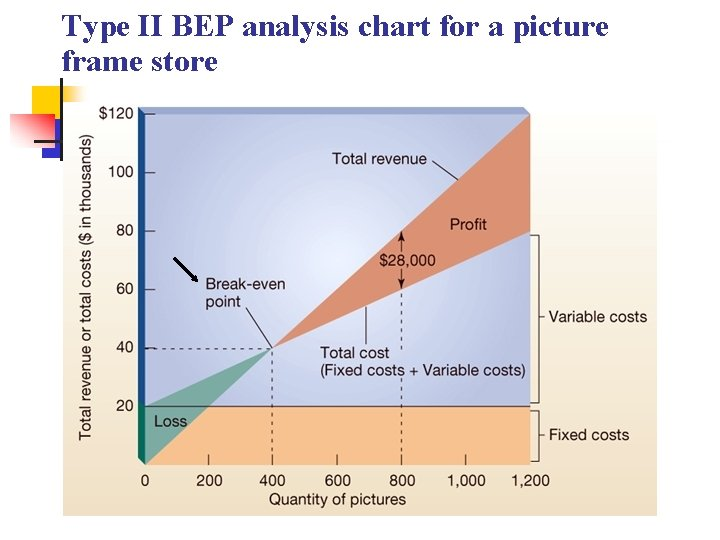Type II BEP analysis chart for a picture frame store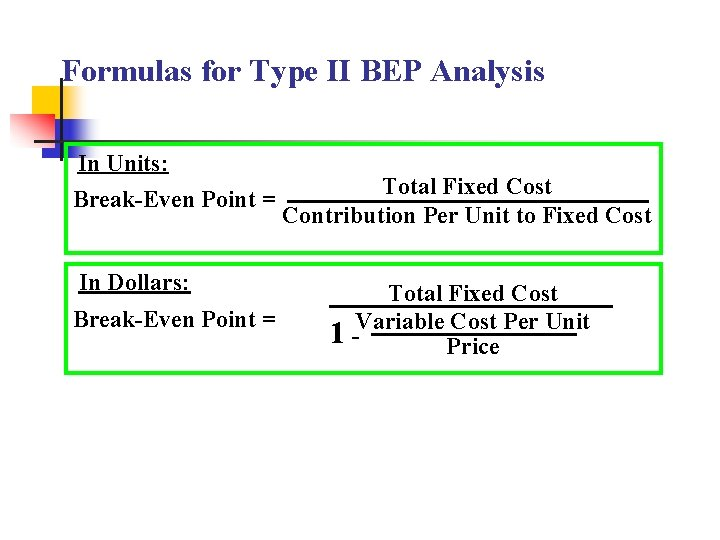Formulas for Type II BEP Analysis In Units: Break-Even Point = In Dollars: Break-Even Point = Total Fixed Cost Contribution Per Unit to Fixed Cost Total Fixed Cost Per Unit 1 -Variable PriceFormulas for Type II BEP Analysis In Units: Break-Even Point = Total Fixed Cost Unit Price – Unit Variable PricePractice Example: Type II BEP in Units n Leeds Manufacturing sells bookcases for \$100 each. They have variable costs of \$50. They want to build a new production line with total fixed cost (TFC)of \$200, 000. What will be the break-even point(BEP) in units to cover this new line? § BEP = TFC / (Unit Price – Unit Variable Cost)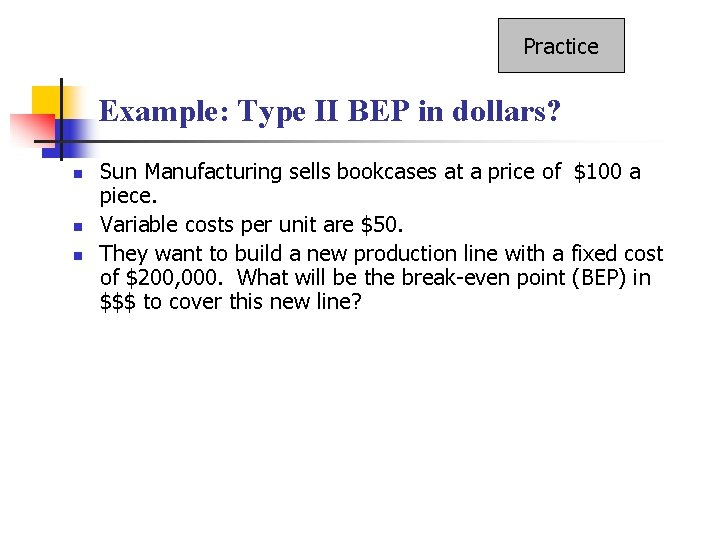Practice Example: Type II BEP in dollars? n n n Sun Manufacturing sells bookcases at a price of \$100 a piece. Variable costs per unit are \$50. They want to build a new production line with a fixed cost of \$200, 000. What will be the break-even point (BEP) in \$\$\$ to cover this new line?Formula for Type II BEP Analysis In Dollars: Break-Even Point = 1 - Total Fixed Cost Variable Cost Per Unit PriceExample: Type II BEP in Dollars Total Fixed Cost BEP (\$) = 1 - Variable Cost per unit /Price \$200, 000 = 1 -. 5 = \$400, 000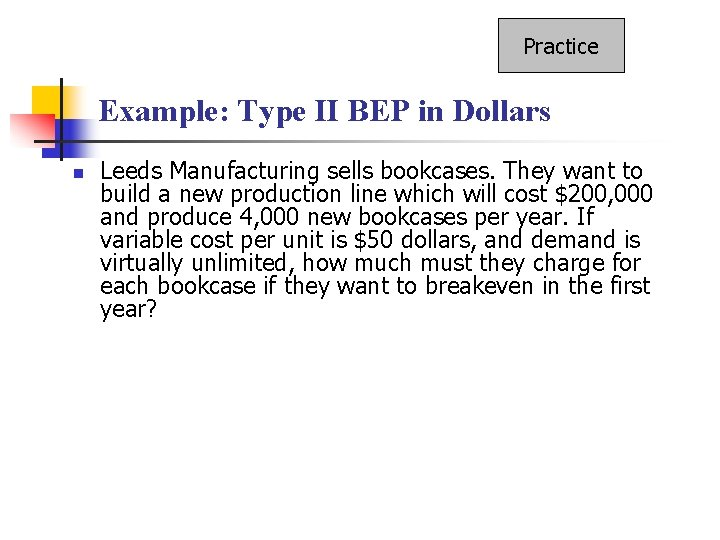Practice Example: Type II BEP in Dollars n Leeds Manufacturing sells bookcases. They want to build a new production line which will cost \$200, 000 and produce 4, 000 new bookcases per year. If variable cost per unit is \$50 dollars, and demand is virtually unlimited, how much must they charge for each bookcase if they want to breakeven in the first year?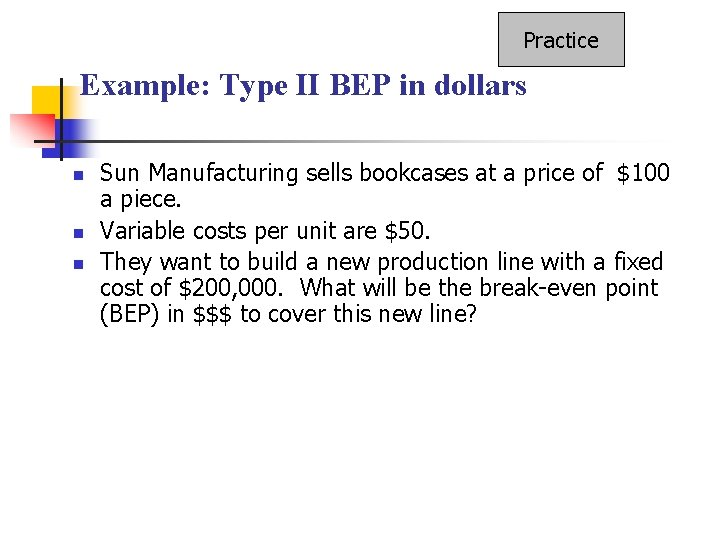Practice Example: Type II BEP in dollars n n n Sun Manufacturing sells bookcases at a price of \$100 a piece. Variable costs per unit are \$50. They want to build a new production line with a fixed cost of \$200, 000. What will be the break-even point (BEP) in \$\$\$ to cover this new line?Types of BEP Type I: BEP of a price change Type II: BEP of a fixed-cost investment BEP Analysis Type III: BEP of the change in variable cost Type IV: BEP of CannibalizationExample: Type III BEP Sun Manufacturing sells bookcases at a price of \$100 a piece. n Variable costs per unit are \$50. § Suppose that the unit variable costs have changed to \$60, what will be the percentage increase in sales volume in order to make the profit remains the same? n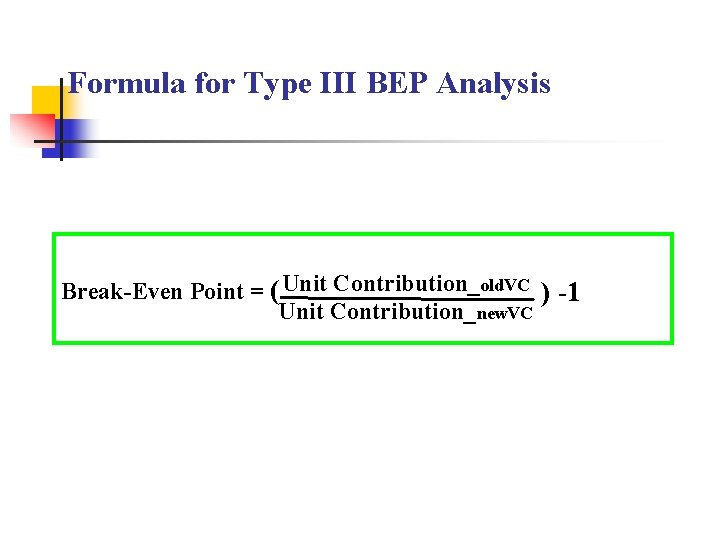Formula for Type III BEP Analysis Break-Even Point = ( Unit Contribution_old. VC ) -1 Unit Contribution_new. VCSolution n n Margin at the old unit variable cost is \$50 Margin at the new unit variable cost is \$40 BEP = n 50 40 -1= 25% To make sure the profit remains the same, the sales volume has to go up by 25%.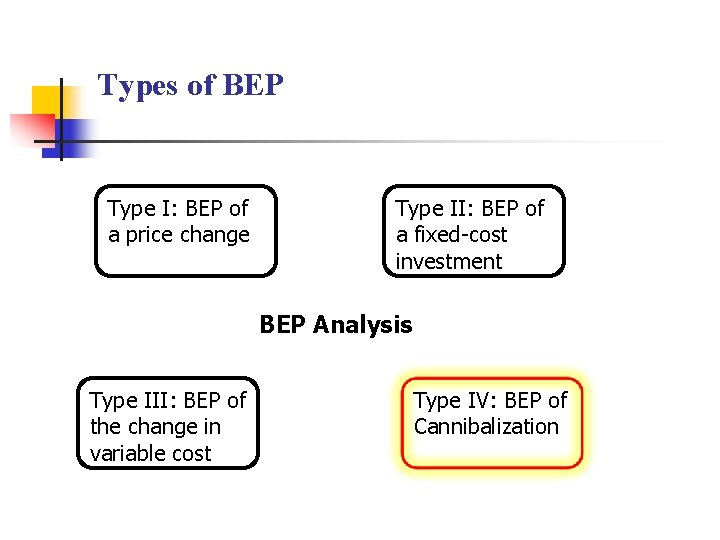Types of BEP Type I: BEP of a price change Type II: BEP of a fixed-cost investment BEP Analysis Type III: BEP of the change in variable cost Type IV: BEP of Cannibalization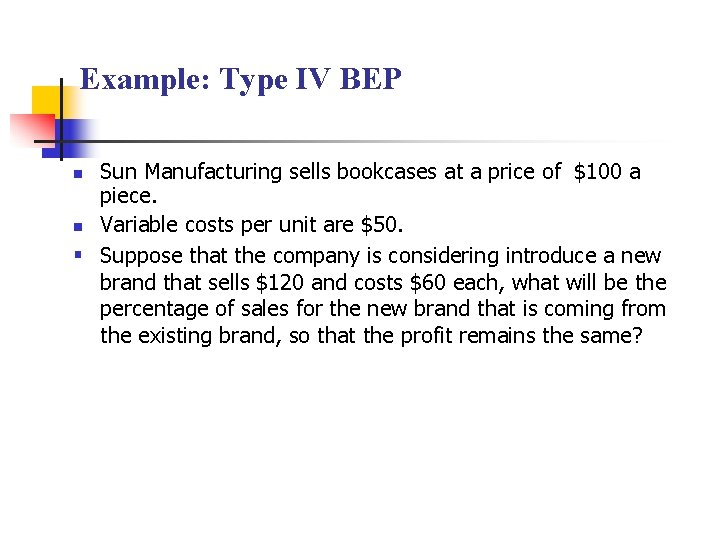Example: Type IV BEP Sun Manufacturing sells bookcases at a price of \$100 a piece. n Variable costs per unit are \$50. § Suppose that the company is considering introduce a new brand that sells \$120 and costs \$60 each, what will be the percentage of sales for the new brand that is coming from the existing brand, so that the profit remains the same? nFormula for Type IV BEP Analysis Break-Even Rate = ( Unit Contribution_new offering Unit Contribution_old offering )Solution n n Margin of the existing product is \$50 Margin of the new product is \$60 50 BEP = 60 n = 83. 3% To make sure the profit remains the same, the new product can take up to 83. 3% of the market share from the existing product.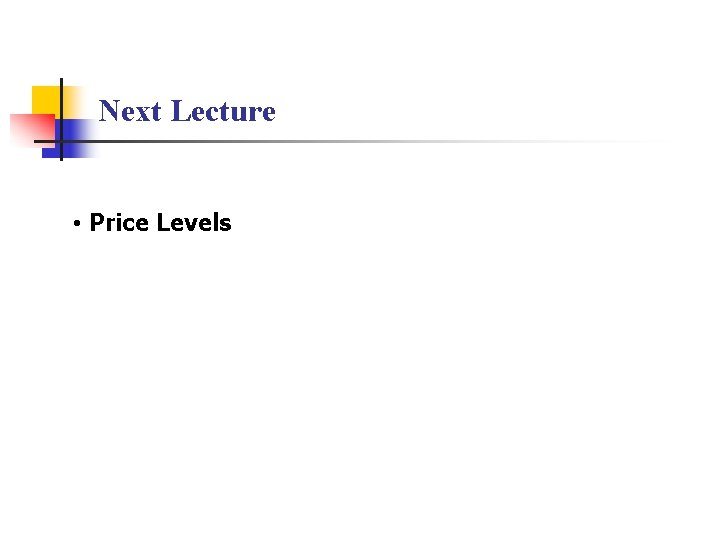Next Lecture • Price Levels